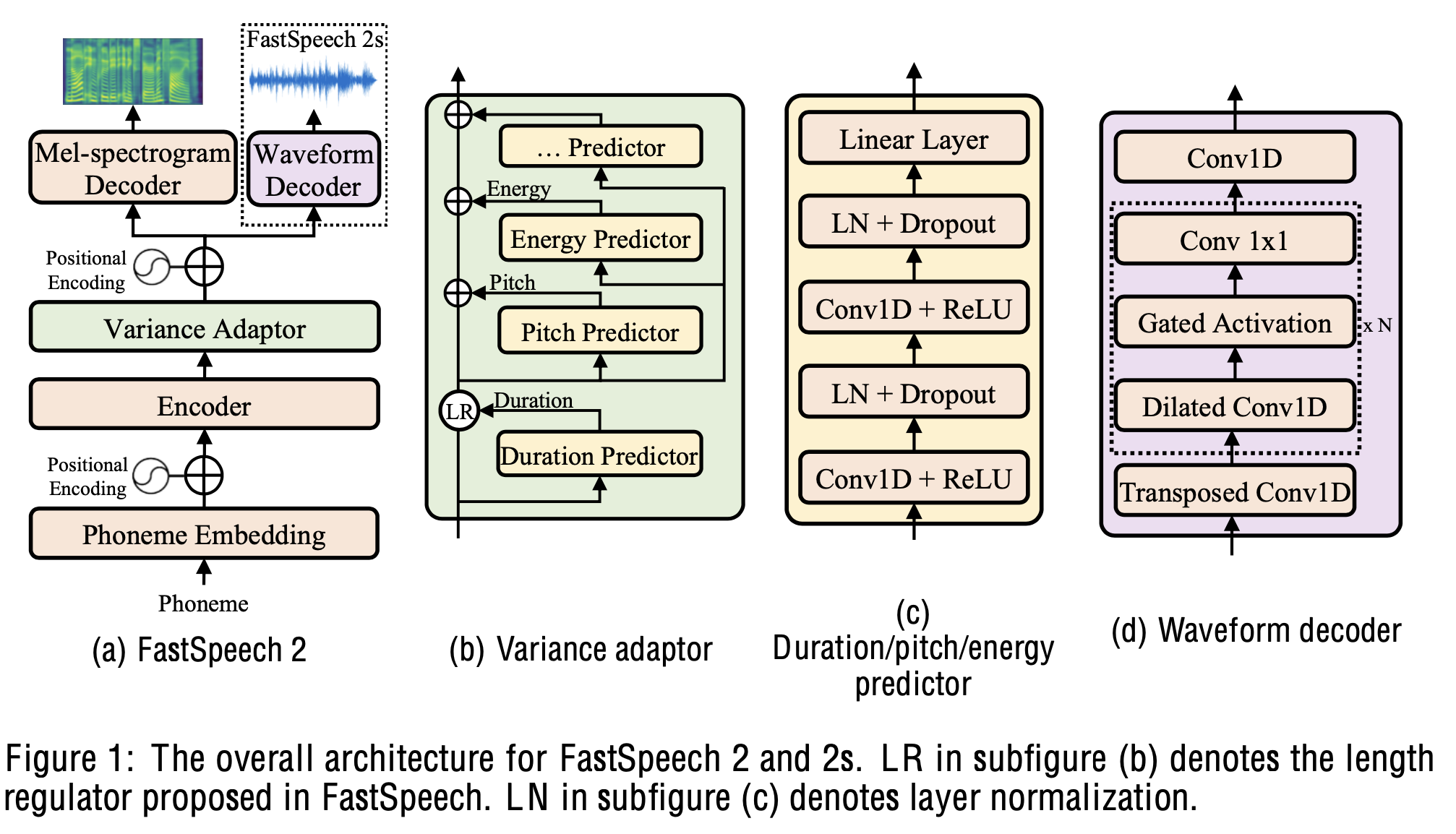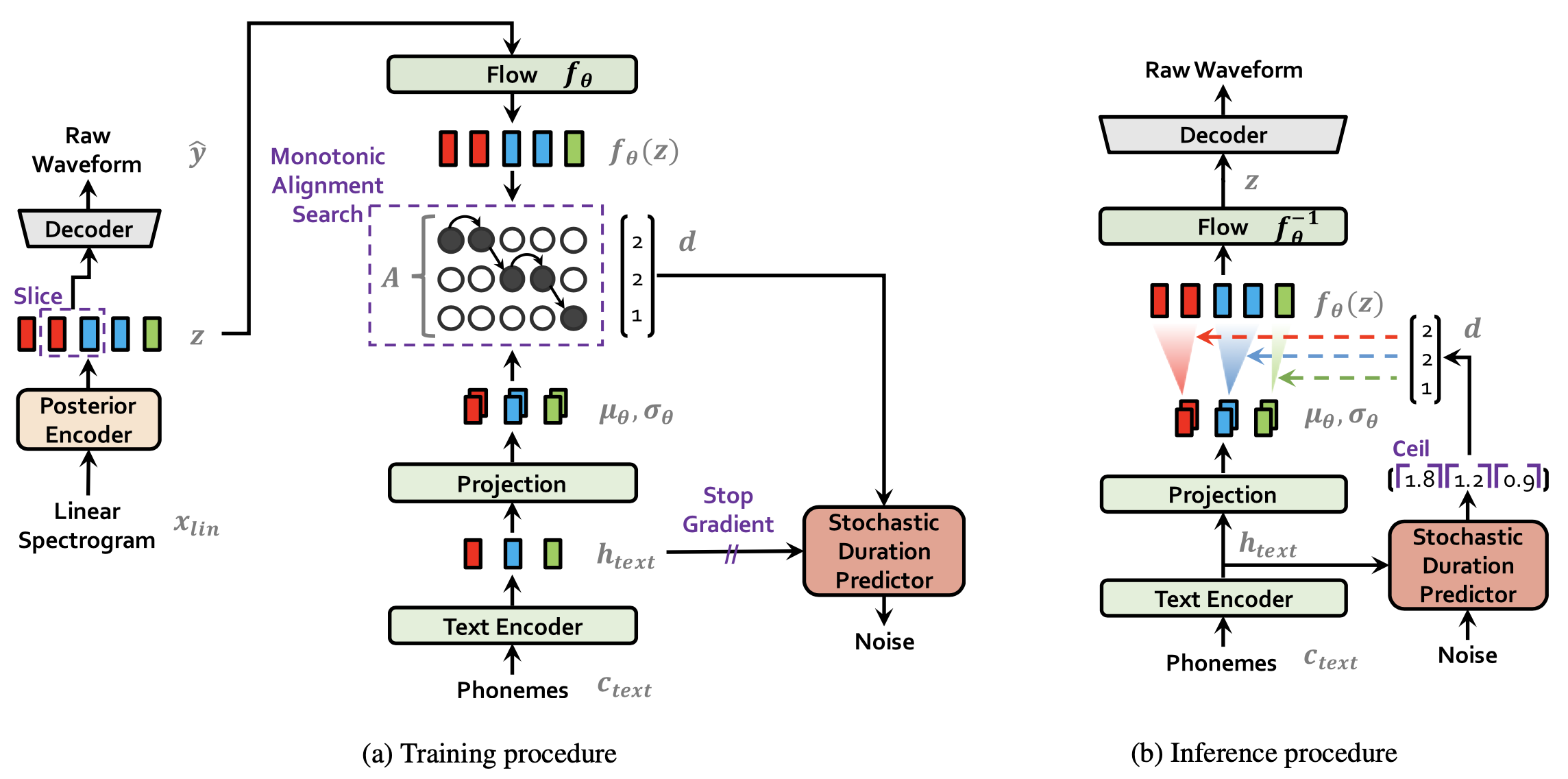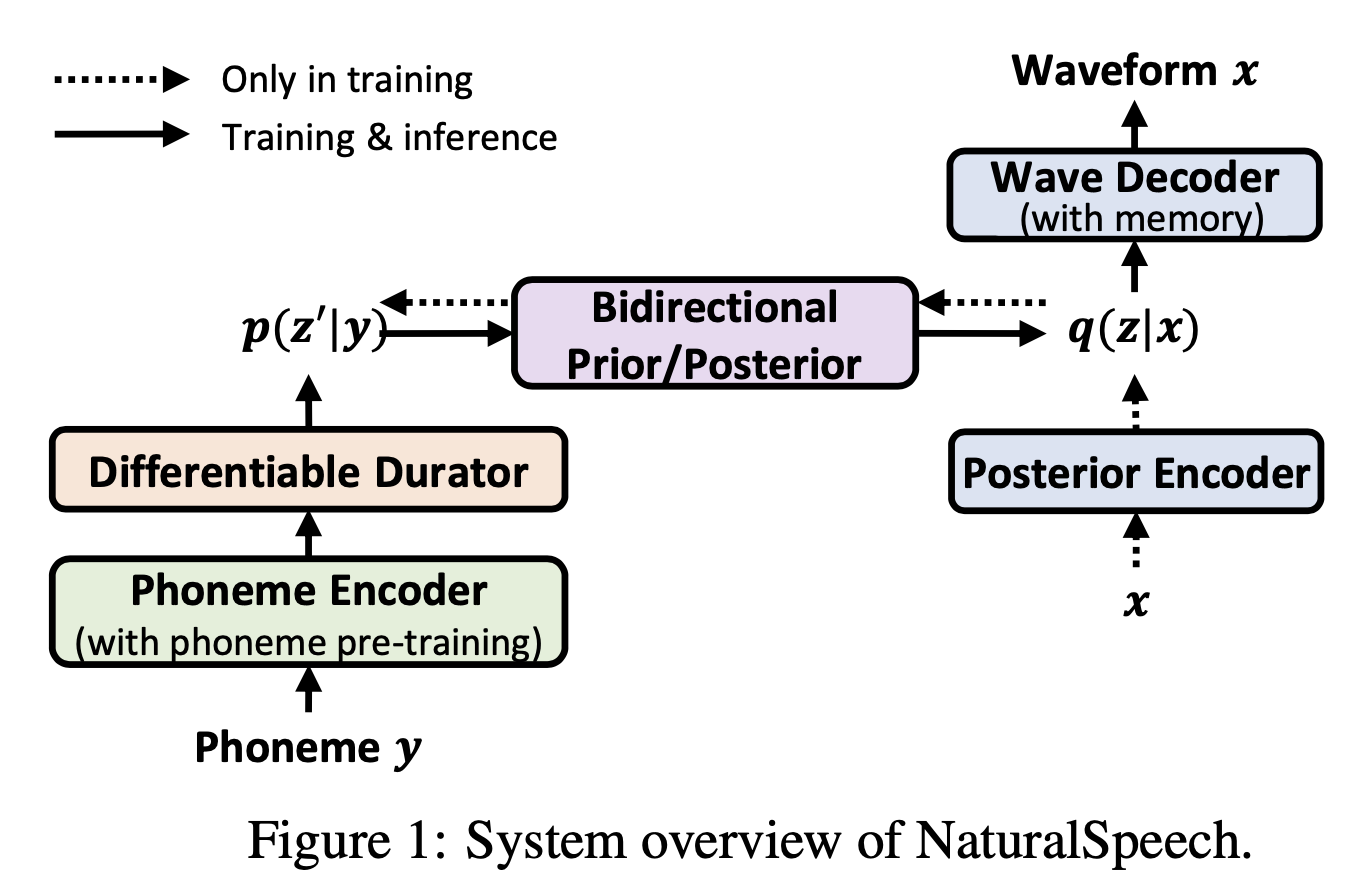# Voice Synthesis

Published:

Speech synthesis with controllable voice is a challenging task.

Recent works propose speech language models for voice cloning, and more details are described in the blog on SpeechLM.

FastSpeech 2(s)^{}Input:

• phonemes
• duration, pitch and energy to mitigate one-to-many mapping in TTS
• (training only) mel-spectrogram, waveform

Data: MFA to align phonemes with frames and get the duration of phonemes

Model: end2end TTS model

• phoneme embedding
• phoneme encoder
• duration predictor
• pitch predictor: frame-level pitch prediction, quantized into 256 bins in log-scale. The discrete pitch is assigned with a pitch embedding when used in mel-spec and waveform generation
• energy predictor: frame-level energy prediction, quantized into 256 bins with learnable energy embeddings.
• two decoders: non-autoregressive decoder
• mel-spectrogram decoder: its output linear layer converts the hidden states into 80d mel-spectrograms.
• waveform decoder: waveform prediction is harder then mel-spectrogram lie its phase information. Waveform decoder is trained with adversarial loss, and used in place of mel-spectrogram decoder during inference for end-to-end TTS.

Training:

• Phonemes together with ground truth duration, pitch and energy are used to predict mel-specotrgram and waveform
• duration, pitch and energy predictors are also trained with the ground truth
• Mean-absolute-error in mel-spectrogram prediction, adversarial loss in waveform prediction.

VITS^{} (Varitioanl Inference for Text-to-Speech)Input:

• phoneme
• (training only) raw waveform

Model:end2end phoneme-to-waveform. $c$: phoneme sequence, $x$: mel-spectrogram, $y$: waveform. $z$: hidden variable, its granularity is the same as spectrogram. High-level is conditional VAE to maximize $p(x|c)$: $c$ is condition phonemes, $x$ is target. VAE learns latent variables $z$ from $c$: $p(z|c)$ to $x$: $p(x|z)$.

• Posterior encoder: encode linear spectrogram with WaveNet residual blocks: $q(z|x_\text{linear-spectrogram})$

• Normalizing flow. It is a stack of affine coupling layers by WaveNet residual blocks. Normalizing flow is applied to $p(z|c,A)$, transforming it to a normal distribution through change-of-variable (note that the transformation is invertible).
• Prior encoder: consisting of a Transformer text encoder (encoding phonemes), a linear projection layer (producing mean and variance). The mean and variance are for the normal distribution which is the result of normalizing flow.

• Alignment estimation between c and z: how long each input phoneme expands to be time aligned with target speech. It serves for providing duration in training stochastic duration predictor, and not used for inference.

• Duration predictor. Although the duration d can be derived from alignment matrix A, the deterministic value cannot reflect human-like speech which changes the speaking rate each time. A stochastic duration predictor has two variables: u (variational dequantization, d-u gives real-valued duration) and v (variational data augmentation, concatenated with d in hidden states).

• Decoder: HiFi-GAN structure to convert representations from Posterior encoder to waveform. (auto-regressive?)

• Discriminator: multi-period discriminator

Training: variational inference, alignment estimation, normalizing flow, adversarial training

• VAE ELBO. VAE is trained with ELBO to maximize the lower bound of $p(x|c)$ given latent variable $z$ via $p(x|z)$ and $p(z|c,A)$ where A is the alignment. Then with normalizing flow, $p(z|c,A)$, is transformed to normal distribution in ELBO.

• Alignment $A$ is searched to maximize ELBO with the constraint of being monotonic and non-skipping.

• Duration predictor’s variational lower bound is defined on latent variables $u$ and $v$. The lower bound of $p(d|c)$ is estimated by $p(u,v|c,d)$ and $p(d-u,v|c)$.

• KL divergence is applied to $\text{flow}(q(z|x_\text{linear-spectrogram}))$ and $p(z|c, A\text{z-and-c})$.

• Latent variable z is upsampled to the waveform domain $\hat{y}$ via a decoder, $\hat{y}$ is transformed into mel-spectrogram. The reconstruction loss is applied to the predicted and gold mel-spectrogram.

• Adversarial and feature matching loss on waveform generated by decoder.

NaturalSpeech^{}Input:

• phonemes
• (training only) raw waveform

Model:

$x$: speech waveform, $y$: phoneme, $z$: latent variable

• Phoneme encoder: encode phoneme sequence $y$ into hidden representations $z’$
• prior distribution: $p(z'|y)$
• Differentiable duration predictor: it takes outputs of phoneme encoder and predict duration of each phoneme.
• learnable upsampling layer: extend hidden sequence from phoneme level to frame level
• start and end index matrix for the phoneme-frame alignment
• additional linear layers: calculate mean and variance of prior distribution $p(z'|y)$
• Posterior encoder: encode waveform $x$ into hidden variables $z$
• posterior distribution: $q(z|x)$
• Bidirectional prior/posterior (flow model)
• backward mapping $z’=f^{-1}(z)$ to reduce posterior: transform posterior $q(z|x)$ to a simpler distribution $q(z'|x)$, and minimize KL-divergence between $q(z'|x)$ and $p(z'|y)$.
• forward mapping $z=f(z’)$ to enhance prior: transform prior $p(z'|y)$ to $p(z|y)$, and minimize KL-divergence between $p(z|y)$ and $q(z|x)$.
• Waveform decoder with memory bank Latent variable z from posterior $q(z|x)$ is used as a query to attend to a memory bank, and the attention results are used for waveform reconstruction. The difference from traditional VAE which uses $z$ to reconstruct $x$ is that posterior $q(z|x)$ does not have to be that complicated and far from prior $p(z|y)$.

Training:

• Waveform reconstruction loss
• Bidirectional prior and posterior loss
• End-to-end loss

Make-A-Voice

Speech units:

(1) Semantic units by HuBERT;

(2) Acoustic tokens by Soundstream.

Training is similar to AudioLM’s coarse-to-fine mechanism, but make-a-voice is built upon a seq2seq model instead of decoder-only language model.

(1) Semantic token extraction. If the input is speech in the case of voice cloning, HuBERT extracts its semantic units; if the input is text in the case of TTS, a text-to-unit transformer learns the units.

(2) Semantic-to-acoustic units. A Transformer concatenates acoustic tokens of speech prompt and semantic units of target as input, and predicts target acoustic tokens. In the application of singing voice synthesis, the input also includes quantized log-f0 which is extracted using YAAPT algorithm.

(3) Unit-based vocoder. Instead of using Soundstream decoder, make-a-voice trains a vocoder to synthesize speech with acoustic tokens from only 3 codebooks.

Application: Voice cloning, text-to-speech synthesis, singing voice synthesis.

References

 Ren Y, Hu C, Tan X, Qin T, Zhao S, Zhao Z, Liu TY. Fastspeech 2: Fast and high-quality end-to-end text to speech. arXiv preprint arXiv:2006.04558. 2020 Jun 8.

 Kim J, Kong J, Son J. Conditional variational autoencoder with adversarial learning for end-to-end text-to-speech. InInternational Conference on Machine Learning 2021 Jul 1 (pp. 5530-5540). PMLR.

 Tan X, Chen J, Liu H, Cong J, Zhang C, Liu Y, Wang X, Leng Y, Yi Y, He L, Soong F. Naturalspeech: End-to-end text to speech synthesis with human-level quality. arXiv preprint arXiv:2205.04421. 2022 May 9.

 Huang R, Zhang C, Wang Y, Yang D, Liu L, Ye Z, Jiang Z, Weng C, Zhao Z, Yu D. Make-A-Voice: Unified Voice Synthesis With Discrete Representation. arXiv preprint arXiv:2305.19269. 2023 May 30.

Tags: